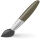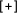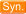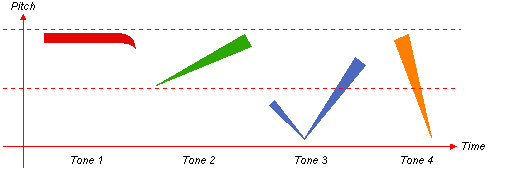# "Derivative"  Chinese-English DictionaryDICTIONARY OPTIONS
 衍生 yǎn shēng to give rise to / to derive / derivative / derivation派生 pài shēng to produce (from sth else) / to derive (from raw material) / derivative衍生物 yǎn shēng wù a derivative (complex product derived from simpler source material)衍生产品 yǎn shēng chǎn pǐn derivative product / derivative (in finance)导数 dǎo shù derivative (math.)次生 cì shēng derivative / secondary / sub-微商 wēi shāng derivative (math.) / business on WeChat 微信[Wei1 xin4] leveraging one's social network / person who operates such a business衍生工具 yǎn shēng gōng jù derivative (finance)保证金 bǎo zhèng jīn earnest money / cash deposit / bail / margin (in derivative trading)派生词 pài shēng cí derivative word偏微分 piān wēi fēn (math.) partial differential / (math.) partial derivative信贷衍生产品 xìn dài yǎn shēng chǎn pǐn credit derivative (in finance)导函数 dǎo hán shù derived function / derivative f' of a function f金融衍生产品 jīn róng yǎn shēng chǎn pǐn financial derivative天气衍生品 tiān qì yǎn shēng pǐn weather derivative第二派生图 dì èr pài shēng tú second derivative map二阶导数技术 second derivative technique第二衍生面 dì èr yǎn shēng miàn second derivative surface不饱和衍生物 unsaturated derivative求导 qiú dǎo to find the derivative (math.)